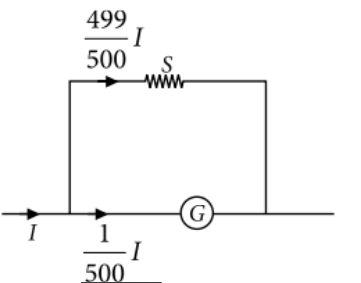Moving coil Galvanometer
Question

# In an ammeter 0.2% of main current passes through the galvanometer. If resistance of galvanometer is G, the resistance of ammeter will be

Moderate
Solution

## Here, resistance of the galvanometer = G Current through the galvanometer, ${I}_{S}=I-{I}_{G}=I-\frac{1}{500}I=\frac{499}{500}I$$\frac{1}{{R}_{A}}=\frac{1}{G}+\frac{1}{S}=\frac{1}{G}+\frac{1}{\frac{G}{499}}=\frac{500}{G}$${R}_{A}=\frac{1}{500}G$

Get Instant Solutions